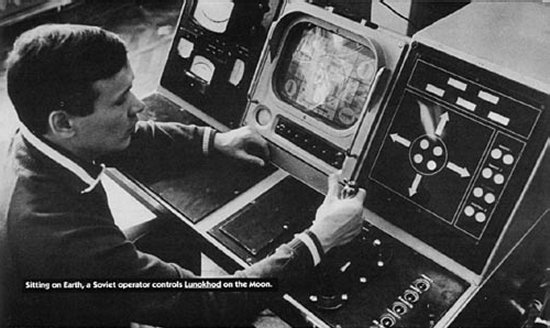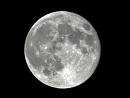# How Much Time The Moon Light Take To Reach Earth?

The distance between Moon and earth = 239,000 Miles.

Light travels at the rate  = 186,000 Miles Per Sec.

time Moon Light Take To Reach Earth = distance/ rate
= 239000/186000
= 5/4

= 1.25 0r 1.3 sec.

thanked the writer.
The moon light does not emit a light source, for the record. It is the sun's light that illuminates the moon need I inform you. With light traveling at just over 186,00 miles per second, the moon is only 250,00 miles from earth. It will only take a little over 1.3 seconds to reach us.
thanked the writer.
If you know the figures of the distance between the Earth and the moon, and the speed of light, it isn't that difficult to figure out:

Distance: 239,000 miles
Speed of Light = 186,000 miles per second

Time = Distance/Rate

Time = 239,000 / 186,000

You should come out around 1.3 seconds... So it takes 1.3 light seconds for light to travel from the Earth to the moon.

thanked the writer.
While the distance of moon to earth's surface is 357000km, moon light is belong to a kind of light, its velocity in air is about 300000km/s, so the time  is  about  1.19s. Hope it helps, good luck
thanked the writer.
1.3 seconds
thanked the writer.
It takes only about one and a quarter seconds for moonlight to reach the earth.thanked the writer.
This depends on the distance from the moon's surface to the earth's surface, which varies in orbit.

If you take an average distance of 350,600,000 m and divide by the speed of light in a vacuum 299,792,458 m/s you get approximately 1.17 seconds.
thanked the writer.
Very very close people, but it's actually 1.26 seconds, so the Moon is 1.26 seconds older than what it appears to be.